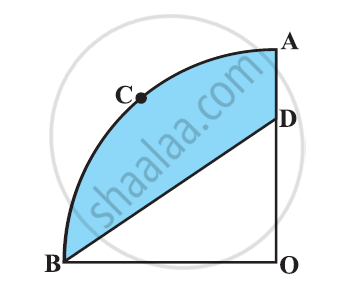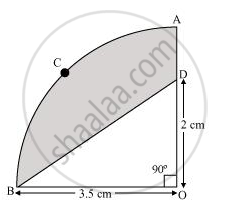# In the given figure, OACB is a quadrant of circle with centre O and radius 3.5 cm. If OD = 2 cm, find the area of the - Mathematics

In the given figure, OACB is a quadrant of circle with centre O and radius 3.5 cm. If OD = 2 cm, find the area of the

[Use Π = 22/7]#### Solution(i) Since OACB is a quadrant, it will subtend 90° angle at O.

Area of quadrant OACB = 90^@/360^@ xx pir^2

=1/4xx22/7xx(3.5)^2 = 1/4xx22/7xx(7/2)^2

= (11xx7xx7)/(2xx7xx2xx2) = 77/8 cm^2

(ii) Area of ΔOBD = 1/2 x OB x OD

= 1/2xx3.5xx2

= 1/2xx7/2xx2

= 7/2 cm^2

Area of the shaded region = Area of quadrant OACB − Area of ΔOBD

= 77/8-7/2

= (77-28)/8

= 49/8 cm^2

Concept: Areas of Combinations of Plane Figures
Is there an error in this question or solution?
Chapter 12: Areas Related to Circles - Exercise 12.3 [Page 237]

#### APPEARS IN

NCERT Class 10 Maths
Chapter 12 Areas Related to Circles
Exercise 12.3 | Q 12 | Page 237
Share